# COP 3402 Systems Software Euripides Montagne University of

• Slides: 19COP 3402 Systems Software Euripides Montagne University of Central Florida Eurípides Montagne University of Central Florida 1COP 3402 Systems Software Predictive Parsing (First and Follow Sets) Eurípides Montagne University of Central Florida 2Outline 1. First set 2. Nullable symbols 3. Follow set 4. Predictive parsing table 5. LL(1) parsing Eurípides Montagne University of Central Florida 3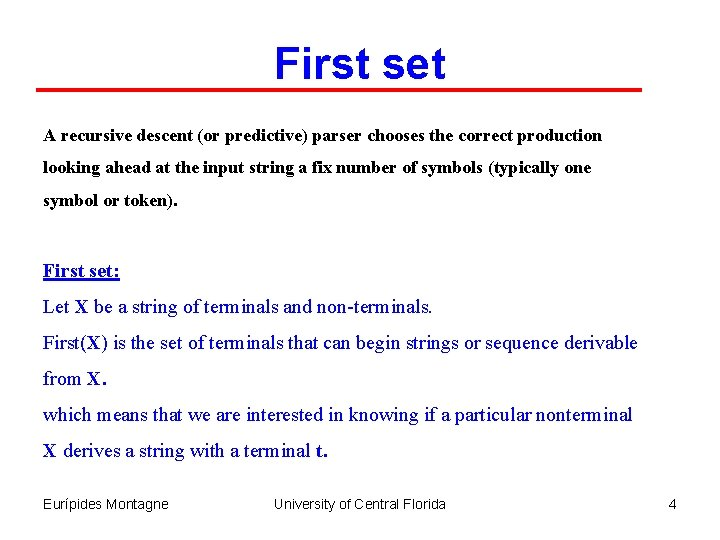First set A recursive descent (or predictive) parser chooses the correct production looking ahead at the input string a fix number of symbols (typically one symbol or token). First set: Let X be a string of terminals and non-terminals. First(X) is the set of terminals that can begin strings or sequence derivable from X. which means that we are interested in knowing if a particular nonterminal X derives a string with a terminal t. Eurípides Montagne University of Central Florida 4First set Definition: FIRST(X) = { t | X ==> * t w for some w} {e | X ==> * e } If X A B C then FIRST(X) = FIRST(A B C) and is computed as follows: If A is a terminal, FIRST(X) = FIRST(A B C) = {A} (for example, if X t B C, FIRST (X) = FIRST( t B C) = { t } Otherwise, if X does not derive to an empty string, FIRST(X) = FIRST(A B C) = FIRST(A). If FIRST(A) contains the empty string then, FIRST(X) = FIRST(A B C) = FIRST(A) – { e } FIRST(BC) Similarly, for FIRST(BC) we have: FIRST(BC) = {B} if B is a terminal, Otherwise, if B does not derive to an empty string, FIRST(BC) = FIRST(B) If FIRST(B) contains the empty string then, FIRST(BC) = FIRST(B) – { e } FIRST(C) And so on… Eurípides Montagne University of Central Florida 5First set Example: S ABC|Cb. B|Ba A da|BC B g|e C h|e FIRST(S) = FIRST(A B C) FIRST(C b B) FIRST(B a) FIRST(A) = FIRST(d a) First(B C) = { d } FIRST(B C) FIRST(B) = FIRST(g) First {e } = {g, e } FIRST(C) = FIRST(h) First {e } = {h, e } Now we can compute: FIRST(BC) = FIRST(B) – {e } {h, e } = {g, e } – { e } {h, e } = {g, h, e } and FIRST(A) = { d } {g, h, e } = { d, g, h, e } Exercise: Compute FIRST(C b B) and FIRST(B a) in order to compute FIRST( S ) Eurípides Montagne University of Central Florida 6First set Example: Given the following expression grammar: E E+T |T T T *F|F F ( E ) | id First(E + T) = { id, ( } Because: E + T T + T F + T id + T E+T T+T F+T (E)+T First(E ) = { id, ( } Because: E T F id E T F (E) Eurípides Montagne University of Central Florida 7Nullable Symbols Nullable symbols are the ones that produce the empty ( e ) string Example: Given the following grammar, find the nullabel symbols and the First set: Z d Y e X Y Z XYZ Y c X a Note that if X can derive the empty string, nullable( X ) is true. X Y e Nullable First Z d X Yes { a, c, e } Z XYZ Y Yes {c, e} Z No {a, c, d}Follow set FOLLOW(A) = { t | S ==> *a A t w for some a, w} Given a production A, Follow( A ) is the set of terminals symbols that can immediately follow A. Example 1: If there is a derivation containing At, then Follow ( A ) = t. Example 2: If the derivation contains A B C t and B and C are nullables, t is in Follow ( A ). Example 3: Given the following grammar: Z d\$ Z XYZ Y e Y c X Y X a Compute First, Follow, and nullable. X Y Z Nullable Yes No Eurípides Montagne First { a, c, e } {c, e} {a, c, d } Follow { a, c, d } {\$ EOF} University of Central Florida 9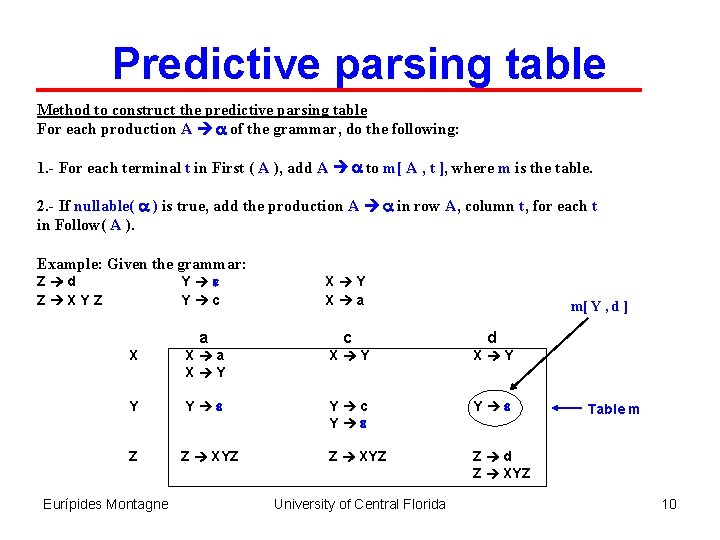Predictive parsing table Method to construct the predictive parsing table For each production A a of the grammar, do the following: 1. - For each terminal t in First ( A ), add A a to m[ A , t ], where m is the table. 2. - If nullable( a ) is true, add the production A a in row A, column t, for each t in Follow( A ). Example: Given the grammar: Y e Y c X Y X a a c d X X a X Y X Y Y Y e Y c Y e Z Z XYZ Z d Z XYZ Eurípides Montagne University of Central Florida m[ Y , d ] Table m 10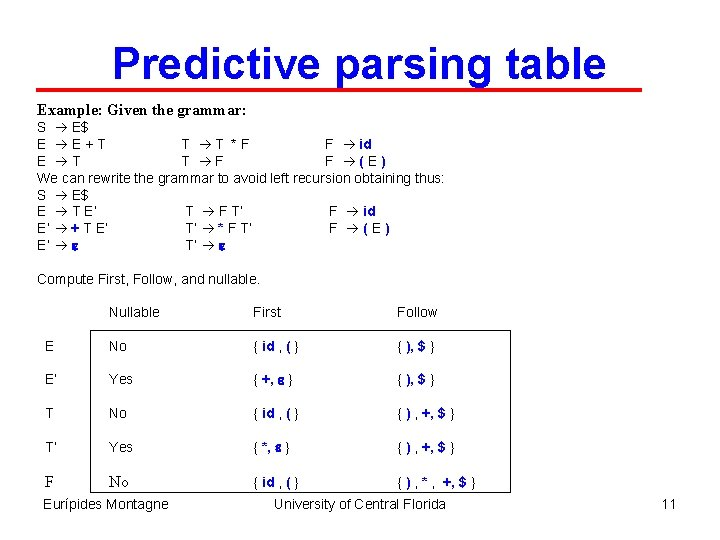Predictive parsing table Example: Given the grammar: S E\$ E E+T T T *F F id E T T F F (E) We can rewrite the grammar to avoid left recursion obtaining thus: S E\$ E T E’ T F T’ F id E’ + T E’ T’ * F T’ F (E) E’ e T’ e Compute First, Follow, and nullable. Nullable First Follow E No { id , ( } { ), \$ } E’ Yes { +, e } { ), \$ } T No { id , ( } { ) , +, \$ } T’ Yes { *, e } { ) , +, \$ } F No { id , ( } { ) , * , +, \$ } Eurípides Montagne University of Central Florida 11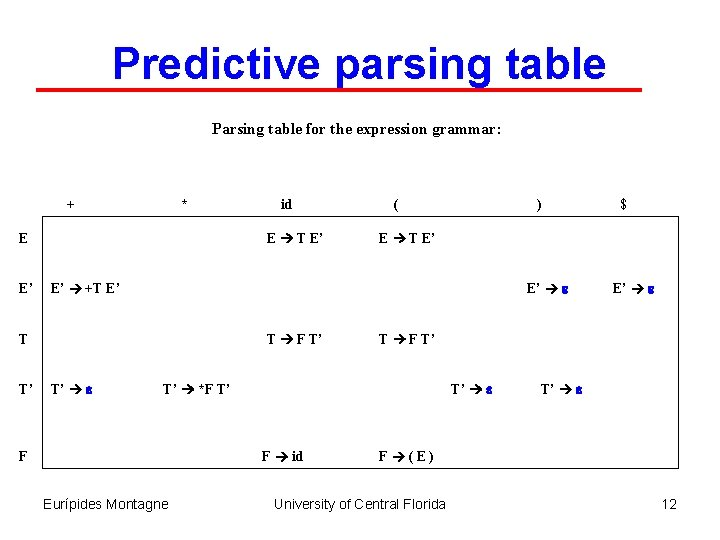Predictive parsing table Parsing table for the expression grammar: + * E T E’ E E’ ( ) E’ e T F T’ T’ e F id Eurípides Montagne E’ e T F T’ T’ *F T’ F \$ E T E’ E’ +T E’ T T’ id T’ e F (E) University of Central Florida 12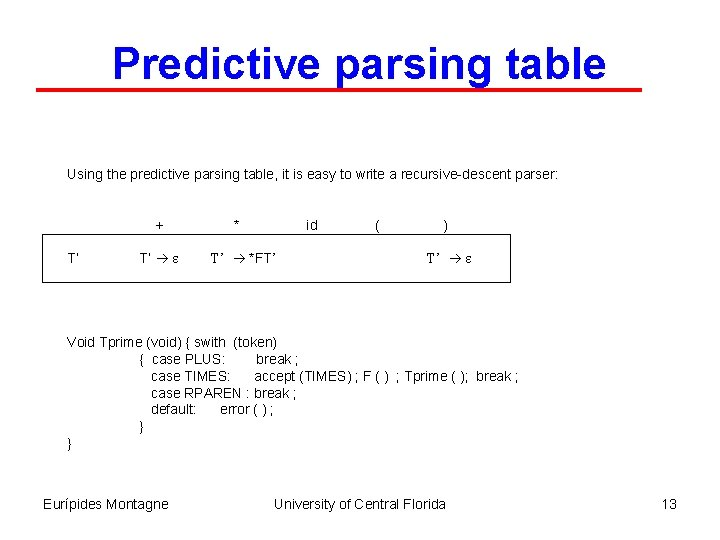Predictive parsing table Using the predictive parsing table, it is easy to write a recursive-descent parser: + T’ T’ e * id T’ *FT’ ( ) T’ e Void Tprime (void) { swith (token) { case PLUS: break ; case TIMES: accept (TIMES) ; F ( ) ; Tprime ( ); break ; case RPAREN : break ; default: error ( ) ; } } Eurípides Montagne University of Central Florida 13Left factoring Another problem that we must avoid in predictive parsers is when two productions for the same non-terminal start with the same symbol. Example: S if E then S S If E then S else S Solution: Left-factor the grammar. Take allowable ending “else S” and e, and make a new production (new non-terminal) for them: S if E then S X X else S X e Grammars whose predictive parsing tables contain no multiples entries are called LL(1). The first L stands for left-to-right parse of input string. (input string scanned from left to right) The second L stands for leftmost derivation of the grammar The “ 1” stands for one symbol lookahead Eurípides Montagne University of Central Florida 14Nonrecursive predictive parsing Example: Given the grammar: S E\$ E T E’ E’ + T E’ E’ e T F T’ T’ * F T’ T’ e F id F (E) With the following First, Follow, and nullable. Nullable First S No { id } E No { id , ( } { ), \$ } E’ Yes {+} { ), \$ } T No { id , ( } { ) , +, \$ } T’ Yes {*} { ) , +, \$ } F No { id , ( } { ) , * , +, \$ } Eurípides Montagne Follow University of Central Florida 15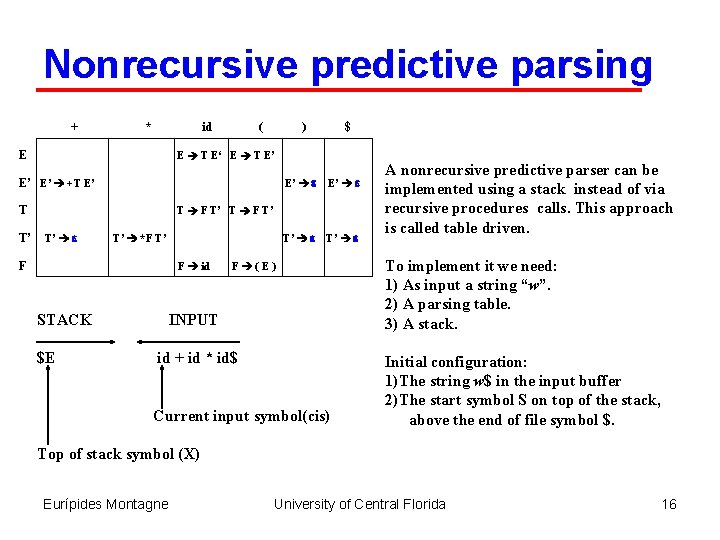Nonrecursive predictive parsing + * id E ( ) E T E‘ E T E’ E’ E’ +T E’ T T’ \$ E’ e T’ e T F T’ T’ e T’ *F T’ F F id STACK \$E F (E) INPUT id + id * id\$ Current input symbol(cis) A nonrecursive predictive parser can be implemented using a stack instead of via recursive procedures calls. This approach is called table driven. To implement it we need: 1) As input a string “w”. 2) A parsing table. 3) A stack. Initial configuration: 1)The string w\$ in the input buffer 2)The start symbol S on top of the stack, above the end of file symbol \$. Top of stack symbol (X) Eurípides Montagne University of Central Florida 16Nonrecursive predictive parsing + * id E ( ) E T E‘ E T E’ E’ E’ +T E’ T T’ \$ E’ e T’ e T F T’ T’ e T’ *F T’ F F id STACK \$E F (E) INPUT id + id * id\$ Current input symbol(cis) Algorithm: Push \$ onto the stack Push start symbol E onto the stack Repeat { /*stack not empty */ If (X = cis) { pop the stack; advance cis to next symbol; } elseif (X is a terminal) error(); elseif (M[X, cis] is an error entry) error(); elseif (M[X, cis] = nonterminal) { pop the stack; push the right hand side of the production in reverse order ; } Let X point to the top of the stack. } until (X = = \$) {accept} Top of stack symbol (X) Eurípides Montagne University of Central Florida 17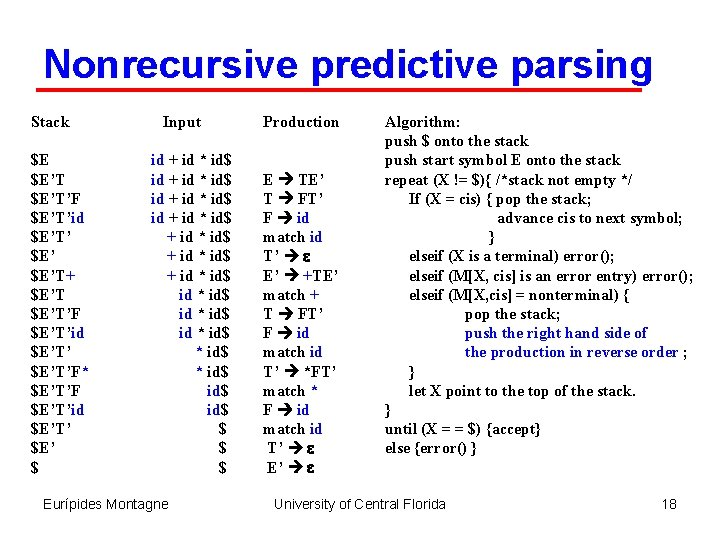Nonrecursive predictive parsing Stack \$E \$E’T’F \$E’T’id \$E’T’ \$E’T+ \$E’T’F \$E’T’id \$E’T’F* \$E’T’F \$E’T’id \$E’T’ \$E’ \$ Input id + id * id\$ + id * id\$ id * id\$ id\$ \$ \$ \$ Eurípides Montagne Production E TE’ T FT’ F id match id T’ e E’ +TE’ match + T FT’ F id match id T’ *FT’ match * F id match id T’ e E’ e Algorithm: push \$ onto the stack push start symbol E onto the stack repeat (X != \$){ /*stack not empty */ If (X = cis) { pop the stack; advance cis to next symbol; } elseif (X is a terminal) error(); elseif (M[X, cis] is an error entry) error(); elseif (M[X, cis] = nonterminal) { pop the stack; push the right hand side of the production in reverse order ; } let X point to the top of the stack. } until (X = = \$) {accept} else {error() } University of Central Florida 18COP 3402 Systems Software Predictive Parsing (First and Follow Sets) The End Eurípides Montagne University of Central Florida 19